# Laying Stepping Stones

## OBJECTIVE: To learn how to change fractions to percents and vice versa

In previous lessons, you learned how to change FRACTIONS to DECIMALS and vice versa, and how to change DECIMALS to PERCENTS and vice versa. Getting to the Point: https://www.geogebra.org/m/wz32mw3y Missing the Point: https://www.geogebra.org/m/vjjpprfm Hurdling the Bar: https://www.geogebra.org/m/ncnyduqx Pointing Left or Right: https://www.geogebra.org/m/ey2tthc4 In this lesson, you're going to learn how to change FRACTIONS to PERCENTS and vice versa. In previous conversions, you only needed one step to do your conversions. Here, however, you cannot do the conversion directly; you need an intermediate step, a stepping stone. To change FRACTIONS to PERCENTS, follow these simple steps: Step 1. Change the fraction to decimal. Step 2. Change the decimal to percent. Examples: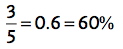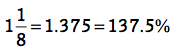To change PERCENTS to FRACTIONS, follow these simple steps: Step 1. Change the percent to decimal. Step 2. Change the decimal to fraction. Examples: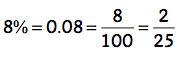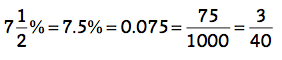If the percent involves a repeating decimal, just use the fraction (change to improper fraction if it's a mixed number) and multiply by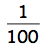. Example: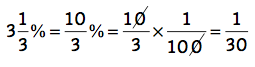Below is a set of problems involving changing fractions to percents and vice versa.

## In this lesson, you learned how to change fractions to percents and vice versa.

In future lessons, you'll learn about other relationships among parts and wholes. Did you have FUN today?